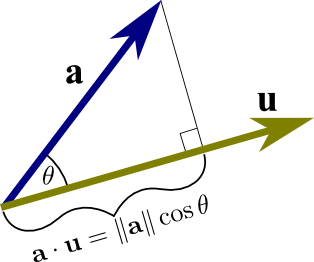# Math Insight

### Image: Dot product as projection onto a unit vectorThe dot product of vectors $\vc{a}$ and unit vector $\vc{u}$ is the projection of $\vc{a}$ onto $\vc{u}$, i.e., $$\vc{a}\cdot \vc{u} = \|\vc{a}\| \cos\theta,$$ where $\theta$ is the angle between $\vc{a}$ and $\vc{u}$. This expression does not involve the magnitude of $\vc{u}$ since it is normalized to be length one.

Image file: dot_product_projection_unit_vector.png

Source image file: dot_product_projection_unit_vector.svg
Source image type: Inkscape SVG